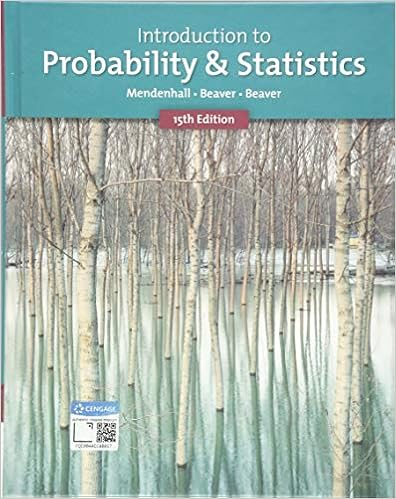# For each of the following questions you must state

• Homework Help
• 5
• 100% (6) 6 out of 6 people found this document helpful

This preview shows page 2 - 4 out of 5 pages.

##### We have textbook solutions for you!
The document you are viewing contains questions related to this textbook.The document you are viewing contains questions related to this textbook.
Chapter 6 / Exercise 19
Introduction to Probability and Statistics
Beaver/MendenhallExpert Verified
For each of the following questions, you must statethe random variable you are using and the distribution assumption you make.(a) What is the probability that an Xmax battery will last at most 800 hours? What is this probabilityfor a Y-cell battery?(b) Assume, two batteries are randomly selected, one Xmax and one Y-cell. What can you say aboutthe differenceDin their lifetimes? It turns out thatDis also Normally distributed. Find expectedvalue and variance and the random variableD.(c) You notice that, on average, Y-cell batteries will last longer than Xmax batteries. But what is theexact probability that the Y-cell batteries will last longer than Xmax batteries?
2
##### We have textbook solutions for you!
The document you are viewing contains questions related to this textbook.The document you are viewing contains questions related to this textbook.
Chapter 6 / Exercise 19
Introduction to Probability and Statistics
Beaver/MendenhallExpert Verified
Stat 330 (Fall 2017): Homework 7Due: October 25, 2017
3. An iid sample ofnobservations is drawn from a population with mean equal to 20 and standard deviationequal to 16. Let¯Xbe the sample mean.(a) Givenn= 64. ApproximateP(¯X <16).(b) Givenn= 64. ApproximateP(16<¯X <23).(c) What is the smallest sample size so that the chance that|¯X-20|>0.5 is at most 0.05?Answer:
•••